Home MATHEMATICS TOPIC 2: FUNCTIONS ~ MATHEMATICS FORM 3

# TOPIC 2: FUNCTIONS ~ MATHEMATICS FORM 3

2025
77### TOPIC 2: FUNCTIONS ~ MATHEMATICS FORM 3

Normally relation deals with matching of elements from the first set called

DOMAIN with the element of the second set called RANGE.
Definitions:
A
function is a relation with a property that for each element in the
domain there is only one corresponding element in the range or co-
domain
Therefore functions are relations but not all relations are functions
The Concept of a Functions Pictorially
Explain the concept of a functions pictorially
Example 1
Which of the following relation are functions?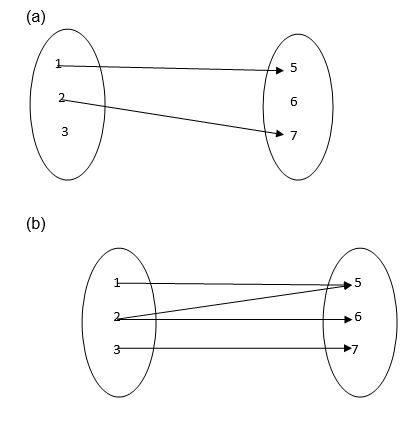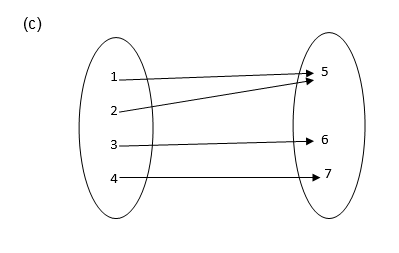Solution
1. It is not a function since 3 and 6 remain unmapped.
2. It is not a function because 2 has two images ( 5 and 6)
3. It is a function because each of 1, 2, 3 and 4 is connected to exactly one of 5, 6 or 7.
Functions
Identify functions
TESTING FOR FUNCTIONS;
If
a line parallel to the y-axis is drawn and it passes through two or
more points on the graph of the relation then the relation is not a
function.
If it passes through only one point then the relation is a function
Example 2
Identify each of the following graphs as functions or not.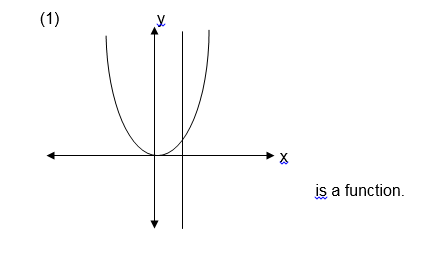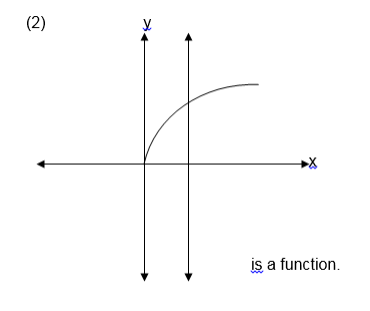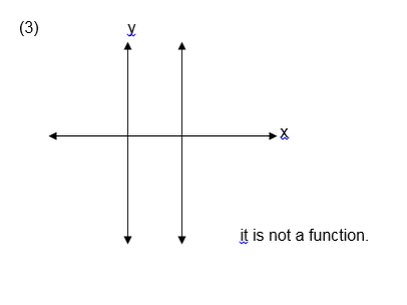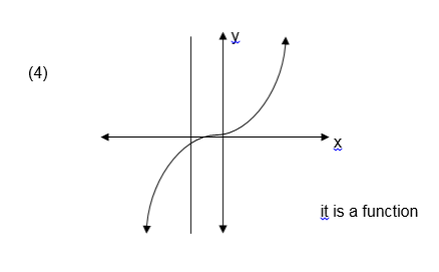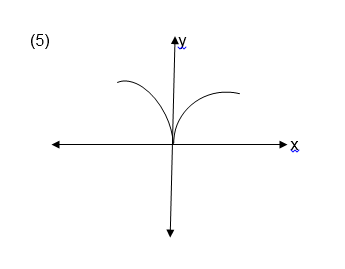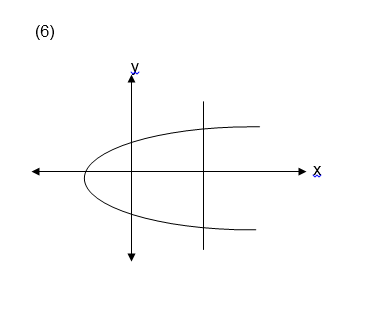Exercise 1
1. Which of the following relations are functions?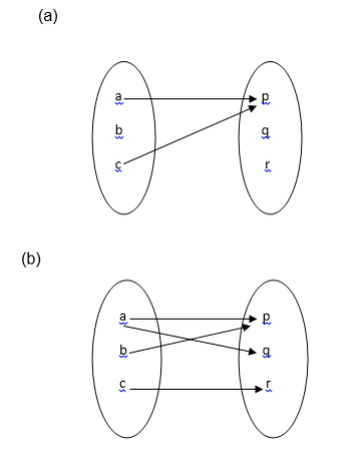2. Let A = {1, 2, 3, 4, 5, 6, 7, 8, 9, 10}
and B ={ 2, 3, 5, 7 }
Draw an arrow diagram to illustrate the relation “ is a multiple of ‘ is it a function ? why?
3. let A = {1,-1 ,2,-2} and
B = {1, 2, 3, 4 } which of the following relations are functions ?
1. { ( x , y ) : x < y }
2. { ( x , y ) : x > y}
3. { ( x , y ) : y = x2}

1. I think this is a real great blog.Thanks Again. Great.

2. Great blog.Thanks Again. Really Cool.

3. I really enjoy the blog post.Really thank you! Keep writing.

4. Enjoyed every bit of your article post. Really looking forward to read more. Much obliged.

5. I think this is a real great blog article.Much thanks again. Awesome.

6. Thank you for your article post.Really looking forward to read more. Much obliged.

7.boucleme

Thank you ever so for you blog post.Much thanks again.

8.gogle

wow, awesome article post. Cool.

9. Thank you ever so for you blog.Thanks Again. Keep writing.

10. Say, you got a nice blog post. Great.

11. Thanks so much for the blog.Really looking forward to read more. Keep writing.